##### Calculus Essentials For DummiesYou can locate a function's concavity (where a function is concave up or down) and inflection points (where the concavity switches from positive to negative or vice versa) in a few simple steps. The following method shows you how to find the intervals of concavity and the inflection points of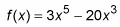1. Find the second derivative of f.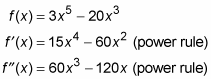2. Set the second derivative equal to zero and solve.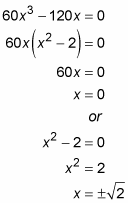3. Determine whether the second derivative is undefined for any x-values.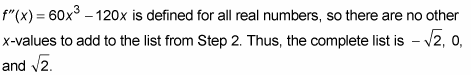Steps 2 and 3 give you what you could call “second derivative critical numbers” of f because they are analogous to the critical numbers of f that you find using the first derivative. But this set of numbers has no special name. In any event, the important thing to know is that this list is made up of the zeros of f′′ plus any x-values where f′′ is undefined.

4. Plot these numbers on a number line and test the regions with the second derivative.

Use -2, -1, 1, and 2 as test numbers.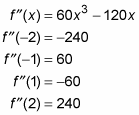Because -2 is in the left-most region on the number line below, and because the second derivative at -2 equals negative 240, that region gets a negative sign in the figure below, and so on for the other three regions.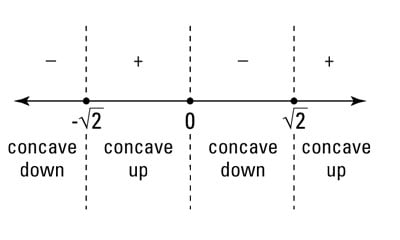A second derivative sign graph

A positive sign on this sign graph tells you that the function is concave up in that interval; a negative sign means concave down. The function has an inflection point (usually) at any x-value where the signs switch from positive to negative or vice versa.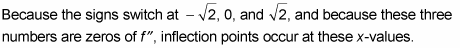If you get a problem in which the signs switch at a number where the second derivative is undefined, you have to check one more thing before concluding that there’s an inflection point there. An inflection point exists at a given x-value only if there is a tangent line to the function at that number. This is the case wherever the first derivative exists or where there’s a vertical tangent.

5. Plug these three x-values into f to obtain the function values of the three inflection points.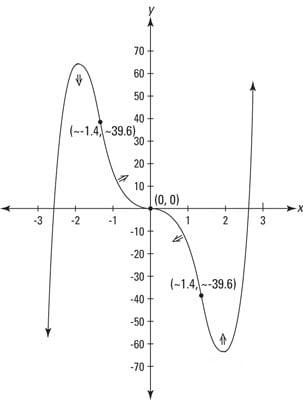A graph showing inflection points and intervals of concavity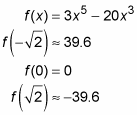The square root of two equals about 1.4, so there are inflection points at about (-1.4, 39.6), (0, 0), and about (1.4, -39.6).